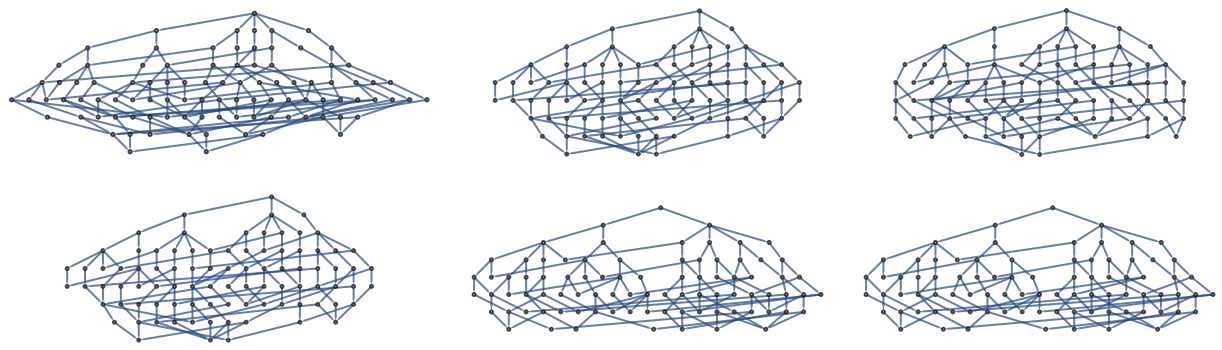Function Repository Resource:

# MultiwayDeletionsGraph

Explore graph traversals while deleting visited components

 ResourceFunction["MultiwayDeletionsGraph"][g] returns a directed acyclic graph which maps all possible traversals of graph g while deleting vertices along the way. ResourceFunction["MultiwayDeletionsGraph"][g,v1] traverses graph g starting only from initial vertex v1. ResourceFunction["MultiwayDeletionsGraph"][g,{v1,v2,…,vn}] traverses graph g starting from n initial vertices vi. ResourceFunction["MultiwayDeletionsGraph"][g,{v1,v2,…,vn},method] allows deleting edges by setting method equals EdgeDelete. ResourceFunction["MultiwayDeletionsGraph"][g,{v1,v2,…,vn},method,max] introduces a cutoff max for cases where the state space graph g is too large to explore efficiently.

## Details

ResourceFunction["MultiwayDeletionsGraph"] takes the same options as Graph.
Vertex names of a ResourceFunction["MultiwayDeletionsGraph"] output depend on a method, either VertexDelete or EdgeDelete:
 w1={{},v1} an initial condition for either method, where v1∈VertexList[g] wn={Sort[{v1,v2,…,vn-1}],vn} with vi∈VertexList[g] and wn∈VertexList[ResourceFunction["MultiwayDeletionsGraph"][g,v1]] wn={Sort[{e1,e2,…,en-1}],vn] withei∈EdgeList[g]andwn∈VertexList[ResourceFunction["MultiwayDeletionsGraph"][g,v1,EdgeDelete]]
Each vertex records an unordered list of vertices or edges visited as well as a current location.
Two vertices wn and wn-1 are adjacent in the output of ResourceFunction["MultiwayDeletionsGraph"] if and only if the following conditions are met, where edge can be either DirectedEdge or UndirectedEdge:
 method=VertexDelete First[wn]==First[wn-1]⋃{vn}&&GraphDistance[g,Last[wn-1],Last[wn]]⩵1 method=EdgeDelete First[wn]==First[wn-1]⋃{edge[vn-1,vn]}&& GraphDistance[g,Last[wn-1],Last[wn]]⩵1
In general, paths through a multiway deletions graph are said to be confluent whenever it is possible to find different valid orderings of the unordered First[wn] which fix the endpoint Last[wn]. Here, "valid" means that, per chosen ordering, each pair of successive vertices wi and wi-1 must satisfy the adjacency criteria.

## Examples

### Basic Examples (2)

Plot two pairs of confluent self-avoiding walks on a 2×2 GridGraph:

 In:=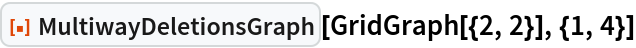Out=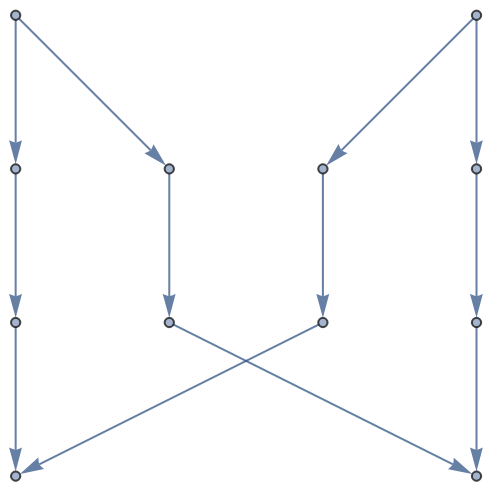Add labels to see which vertices where visited when:

 In:=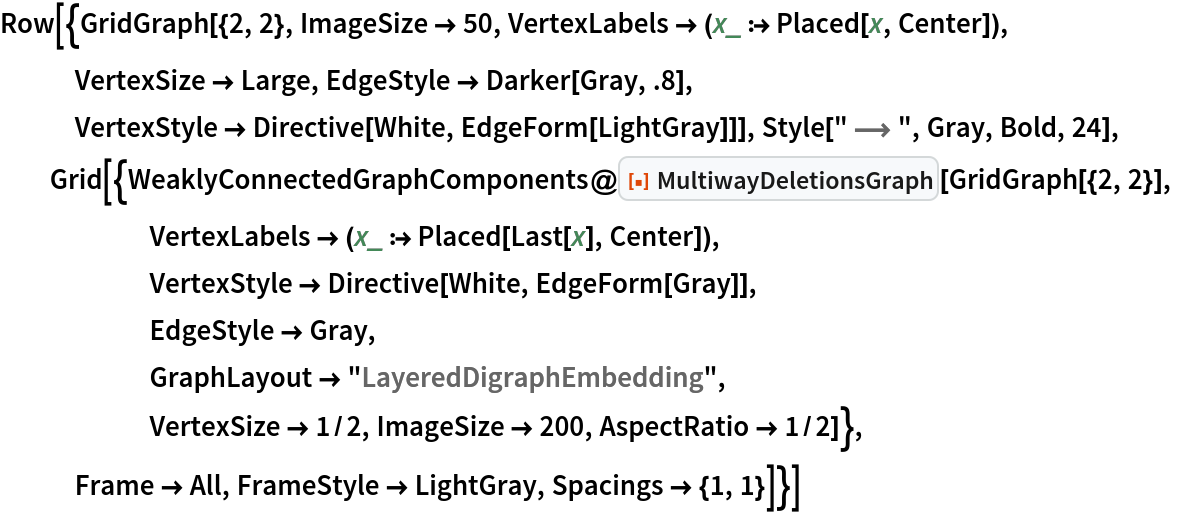Out=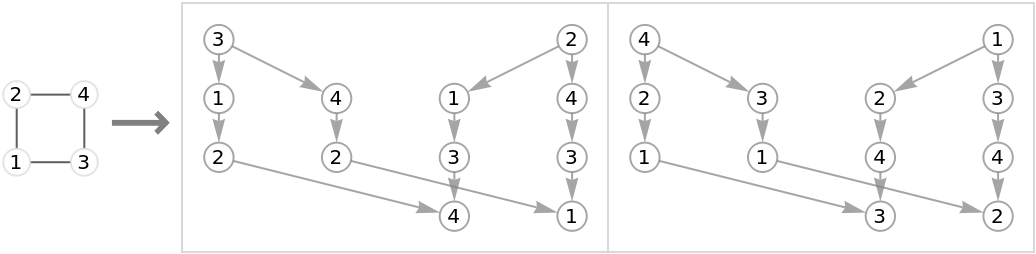Graph self-avoiding walks on a 3×3 grid:

 In:=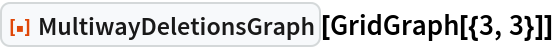Out=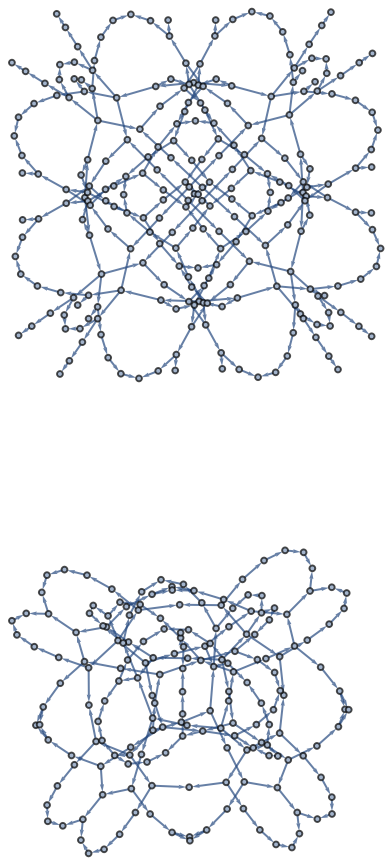Only compute the subgraph starting from vertex 5:

 In:=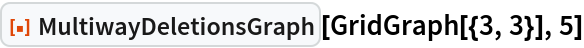Out=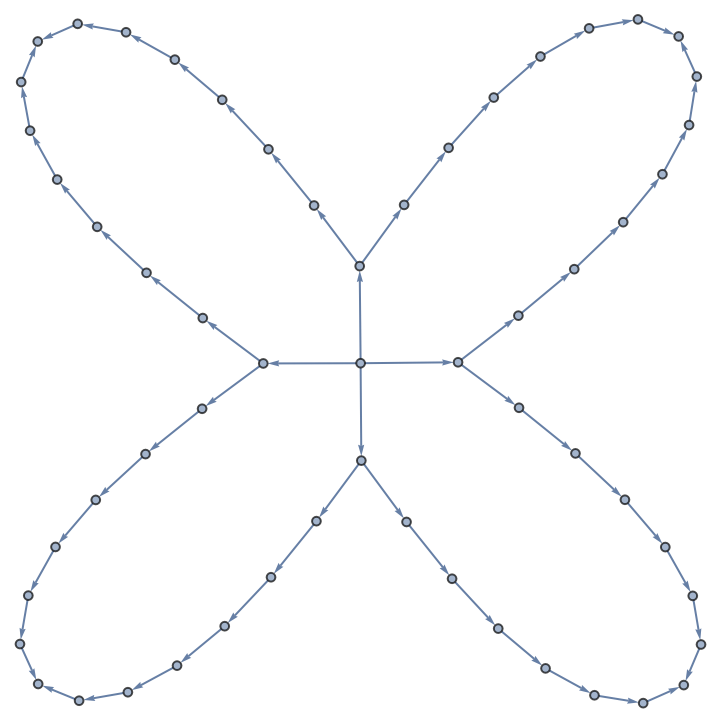Decorate the graph with publication-quality icons:

 In:=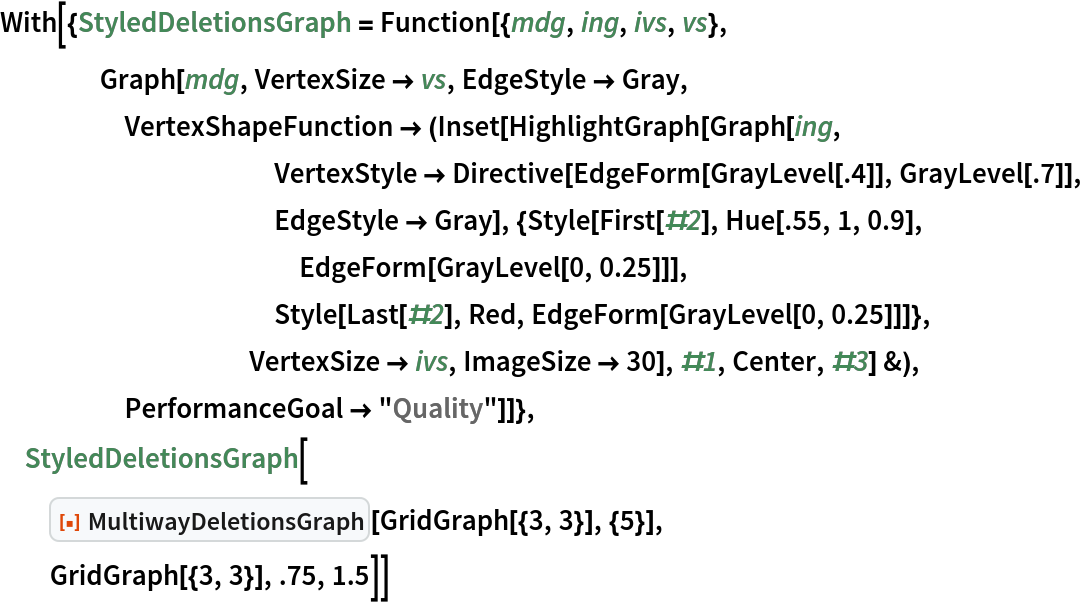Out=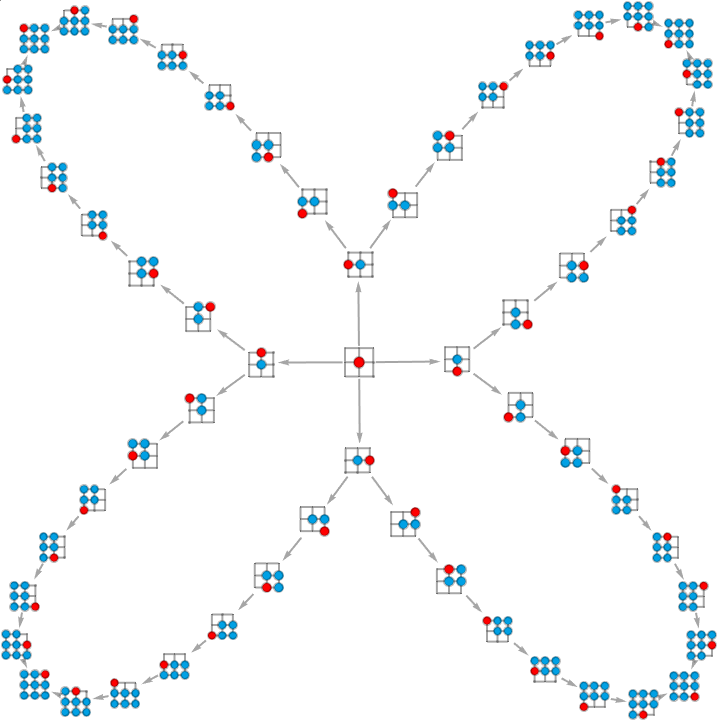Allow walks from vertex 5 to intersect on vertices, but not on edges:

 In:=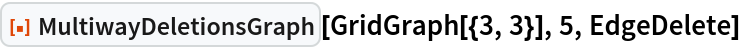Out=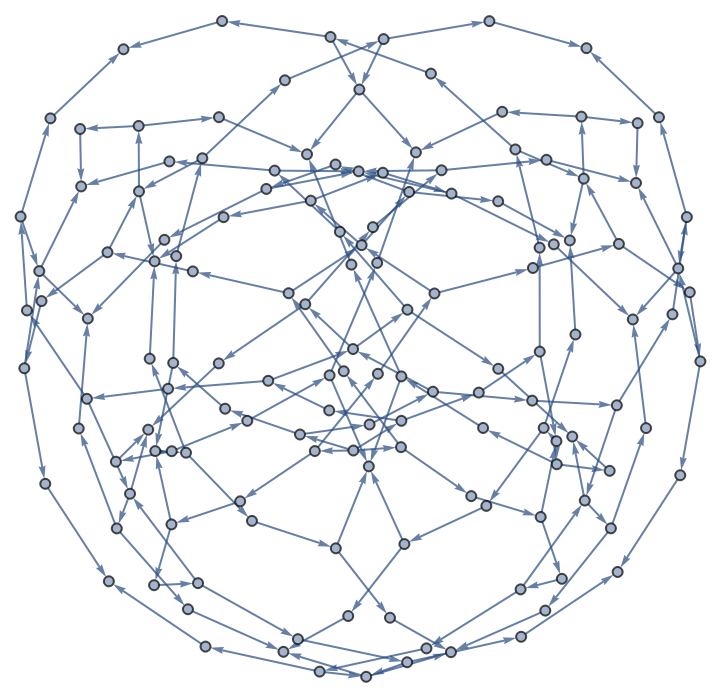Again, decorate the graph with publication-quality icons:

 In:=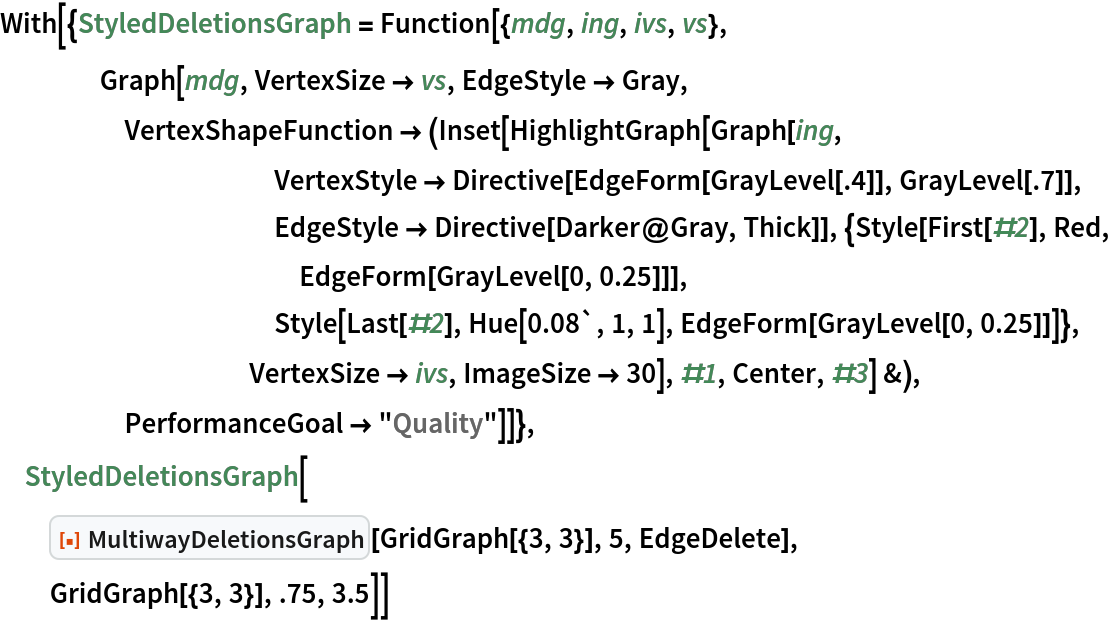Out=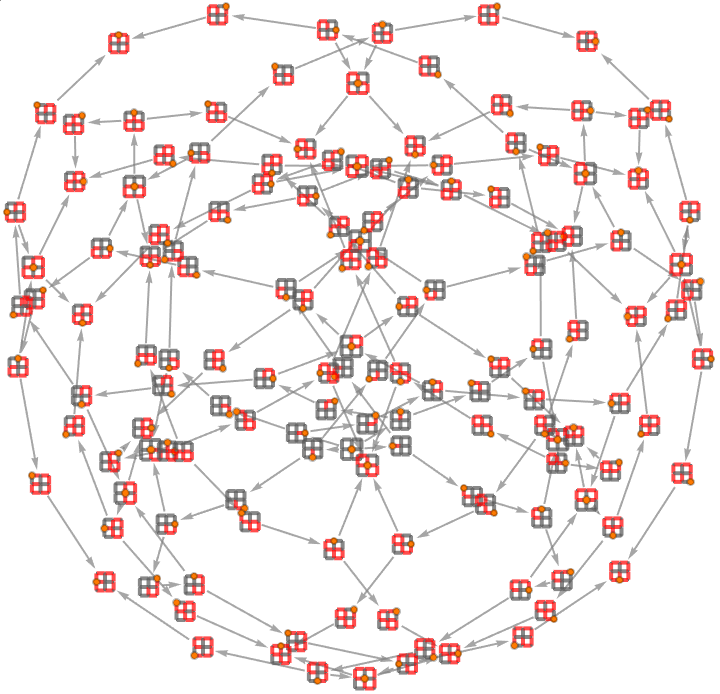### Scope (1)

Test graph transformations on random inputs:

 In:=Out=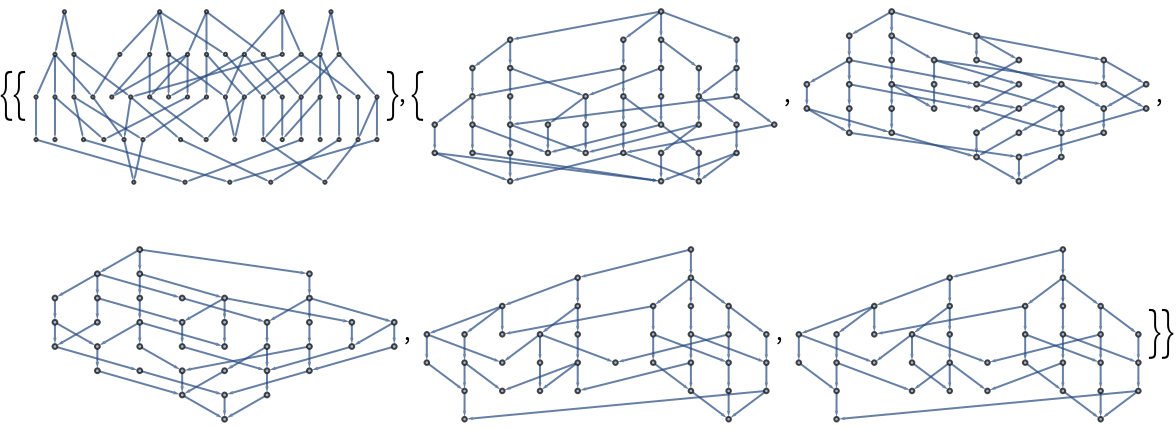### Properties and Relations (1)

When inputting a directed acyclic graph, the output is always a TreeGraph:

 In:=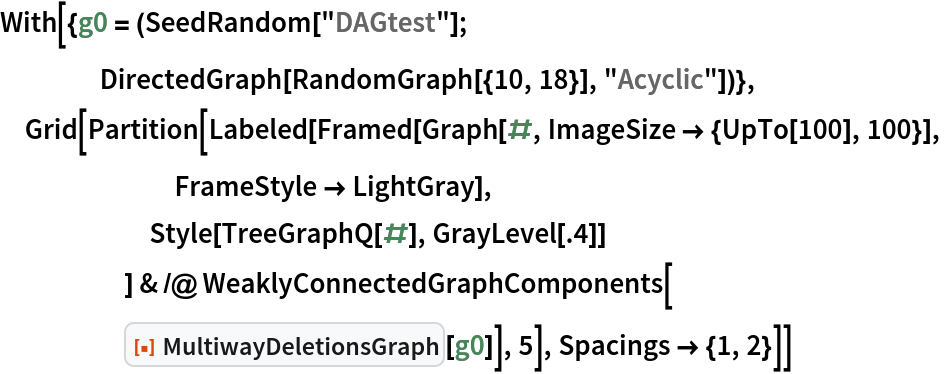Out=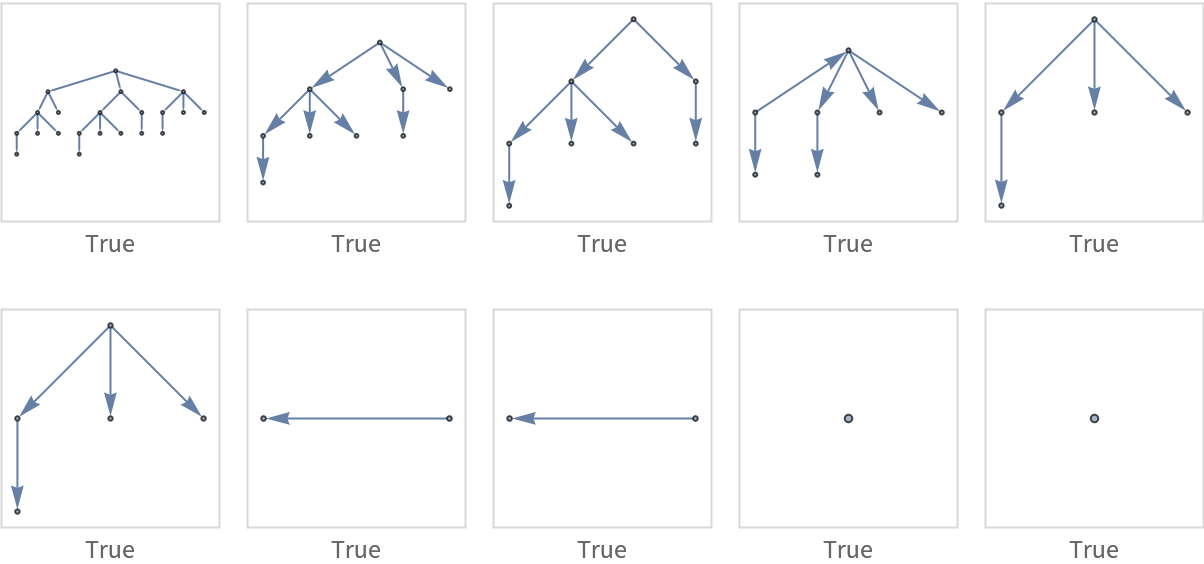### Possible Issues (1)

MultiwayDeletionsGraph automatically deduplicates edges:

 In:=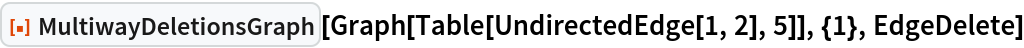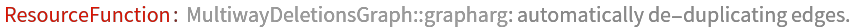Out=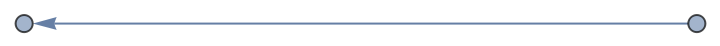### Neat Examples (2)

Graph non-self-intersecting walks along the edges of a cube:

 In:=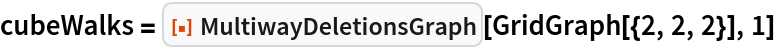Out=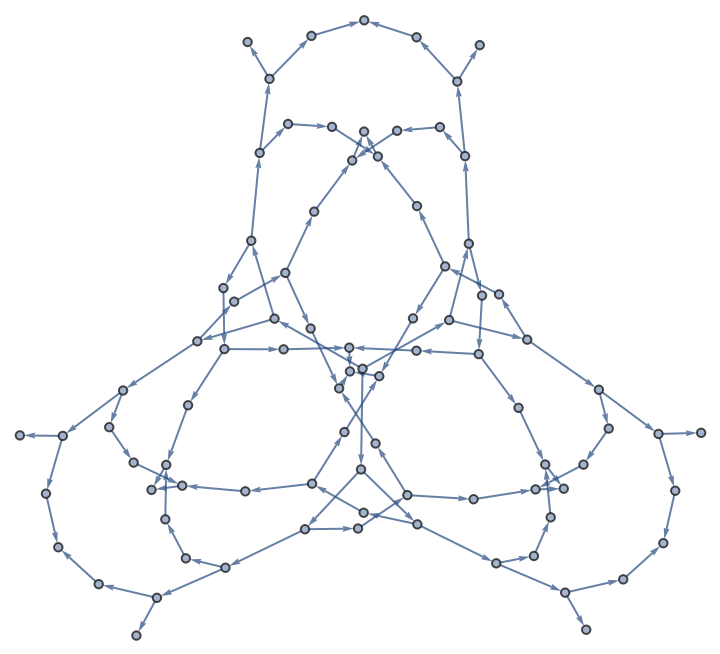Find the out vertices associated with Hamiltonian cycles:

 In:=Out=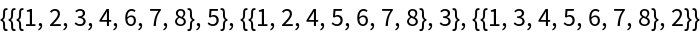Count the number of directed Hamiltonian cycles:

 In:=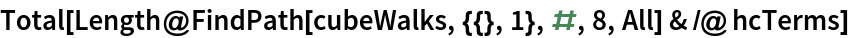Out=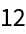Solve Euler's bridges of Königsberg problem by computing all possible paths:

 In:=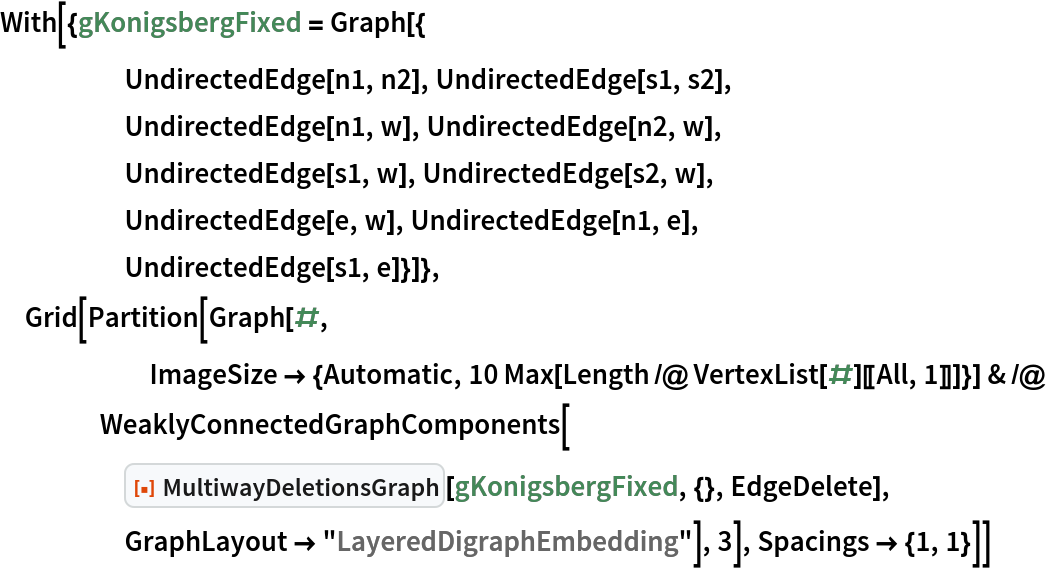Out=